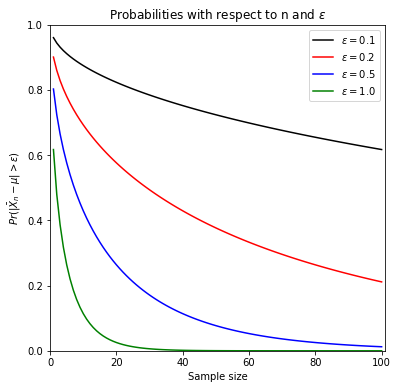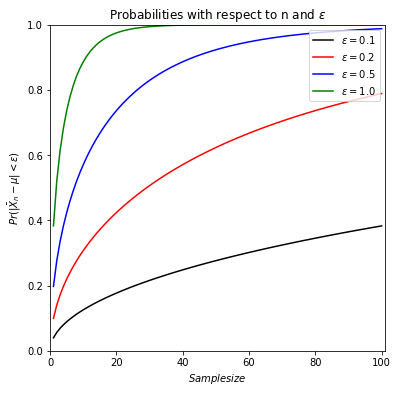# Consistency of Sample Mean¶

Assume that we have a random sample of size n from a population with $$N(\mu, \sigma^2)$$. Let’s investigate how the probability $$Pr(|\bar{X}_{n}-\mu| > \epsilon)$$ changes with respect to n and $$\epsilon$$ and, when $$\sigma^2 = 4$$.

Note that due to symmetry of normal distribution, $$Pr(|\bar{X}_{n}-\mu| > \epsilon) = 2 Pr\big(Z > \frac{\epsilon}{\sigma /\sqrt n}\big)$$.

# import required libraries
import numpy as np
import scipy.stats as stats
import matplotlib.pyplot as plt
from IPython.display import display

def xbar_consistency(eps, col):

#fixed
sigma = 2

fig, ax = plt.subplots(figsize = (6, 6))

for e, cl in zip(eps, col):
samp_size = np.arange(1, 101, 1)
#calculate the probability under given settings
prob = 2*(1-stats.norm.cdf(x = e*np.sqrt(samp_size)/sigma, loc = 0, scale = 1))
#plot
plt.plot(samp_size, prob, c = cl, label = r'$\epsilon=%.1f$' % (e))

plt.xlim(0, 101)
plt.ylim(0, 1)
plt.xlabel(r'Sample size')
plt.ylabel(r'$Pr(|\bar{X}_{n}-\mu| > \epsilon)$')
plt.title('Probabilities with respect to n and $\epsilon$')
plt.legend(loc='upper right')
plt.show()


eps_values = [0.1, 0.25, 0.5, 1]
col_values = ['black', 'red', 'blue', 'green']
xbar_consistency(eps = eps_values, col = col_values)For a given $$\epsilon$$ value, the probability $$Pr(|\bar{X}_{n}-\mu| > \epsilon)$$ forms a sequence and it decreases towards zero as we increase the sample size $$n$$. When we change the value of maximum difference, $$\epsilon$$.

We can also investigate how the complementary probability, namely, $$Pr(|\bar{X}_{n}-\mu| < \epsilon)$$ changes with respect to n and $$\epsilon$$ and , when $$\sigma^2 = 4$$.

def xbar_consistency_toone(eps, col):

#fixed
sigma = 2

fig, ax = plt.subplots(figsize = (6, 6))

for e, cl in zip(eps, col):
samp_size = np.arange(1, 101, 1)
#calculate the probability under given settings
prob1 = 2*(1-stats.norm.cdf(x = e*np.sqrt(samp_size)/sigma, loc = 0, scale = 1))
prob = 1-prob1
#plot
plt.plot(samp_size, prob, c = cl, label = r'$\epsilon=%.1f$' % (e))

plt.xlim(0, 101)
plt.ylim(0, 1)
plt.xlabel(r'$Sample size$')
plt.ylabel(r'$Pr(|\bar{X}_{n}-\mu| < \epsilon)$')
plt.title('Probabilities with respect to n and $\epsilon$')
plt.legend(loc='upper right')
plt.show()


eps_values = [0.1, 0.25, 0.5, 1]
col_values = ['black', 'red', 'blue', 'green']
xbar_consistency_toone(eps = eps_values, col = col_values)Similarly, for a given $$\epsilon$$ value, the probability $$Pr(|\bar{X}_{n}-\mu| < \epsilon)$$ forms a sequence and it increases towards one as we increase the sample size $$n$$. For a given sample size, when we change the value of maximum difference, $$\epsilon_1$$ to $$\epsilon_2$$ ($$\epsilon_1 < \epsilon_2$$),we see that $$Pr(|\bar{X}_{n}-\mu| < \epsilon_1) < Pr(|\bar{X}_{n}-\mu| < \epsilon_2)$$ which makes sense that falling into a wider a wider interval results in a higher probability.